# BETWEEN BEAUTY AND REGULARITY

Hans Walser, Basel University, Switzerland

hwalser@bluewin.ch

Abstract: Cutting away a rhombus from a regular pentagon, the leftover will be a semi-regular pentagon. Using this semi-regular pentagon as tile, we can build various ornaments, tilings, crystallographic patterns, and spirals. We will find also a semi-regular not convex dodecahedron. The regular and the semi-regular dodecahedron together can fill the space without gaps or overlapping.

## The semi-regular pentagon

The regular pentagon is a bad guy.

We can’t use it for a tiling of the plane. There occurs a gap of 36° (Fig. 1c) (Grünbaum and Shephard 1987, Frontispiece).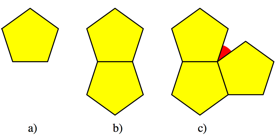Fig. 1: No tiling with regular pentagons

Now we modify the regular pentagon (Fig. 2a). We flip down a vertex (Fig. 2b). The remaining part will be our semi-regular pentagon (Fig. 2c). The semi-regular pentagon has equal sides, but not equal angles.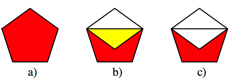Fig. 2: The semi-regular pentagon

Though our semi-regular pentagon has some defects compared to the regular pentagon, it has also some advantages.

## Combination with the regular pentagon

We find a tiling with a combination of regular and semi-regular pentagons (Fig. 3a).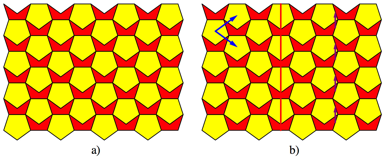Fig. 3: Tiling with pentagons and semi-regular pentagons

The tiling contains translational symmetries, indicated by blue arrows in the figure 3b, axial symmetries (red) and glide-reflection symmetries (purple).

The figure 4 depicts an example with a fivefold rotational symmetry.Fig. 4: Fivefold rotational symmetry

## Frieze patterns and tilings

Nevertheless we may build frieze patterns and tilings just out of our semi-regular pentagon.

The figure 5 depicts a frieze pattern. With respect to the colors it has translational symmetry only. Without respect to the colors we have additionally a glide-reflection symmetry.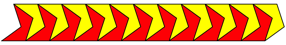Fig. 5: Frieze pattern

The tiling of the figure 6a is just an overlapping of the frieze pattern of the figure 5. In this figure we may get the victim of an optical illusion. Are the horizontal lines parallel?

The figure 6b depicts a more elegant tiling.

What’s the difference between the figures 6c and 6d?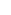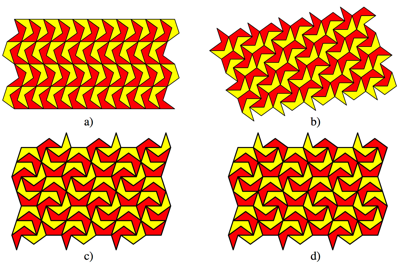Fig. 6: Tilings

## Rings and spirals

The figure 7a gives a constellation with concentric rings. The figure 7b is basically the same, but the colors are exchanged such that there are always two different colors at an edge.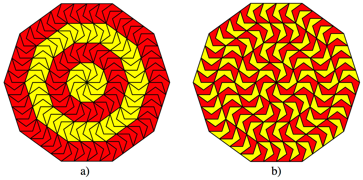Fig. 7: Concentric rings

First we see nothing special in the figure 8a. But a closer look reveals a spiral (Fig. 8b). The width of the spiral is constant, so we have an Archimedean spiral.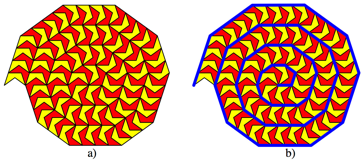Fig. 8: Spirals

The figure 9 depicts two and ten Archimedean spirals respectively.Fig. 9: More spirals

## In the three-dimensional space

With twelve regular pentagons as faces we can build the regular dodecahedron (Fig. 10a). Together with the regular tetrahedron, the cube, the octahedron, and the icosahedron it belongs to the five regular Platonic solids.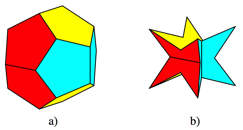Fig. 10: Regular and semi-regular dodecahedron

With twelve semi-regular pentagons we can build the semi-regular dodecahedron (Fig. 10b).

## Relation to the cube

The semi-regular dodecahedron fits obviously in a cube (Fig. 11b). But we can also draw a cube on the faces of the regular dodecahedron (Fig. 11a).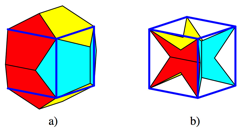Fig. 11: Relation to the cube

The regular dodecahedron may be seen as a compound of a cube with a hip roof on every face. And surprisingly the semi-regular dodecahedron is just the leftover when we cut away a hip roof at every side of the cube. So we have related construction principles. The regular dodecahedron is positive, the semi-regular dodecahedron negative. The hip roof excess of the regular dodecahedron corresponds to the hip roof deficit of the semi-regular dodecahedron.

The figure 12 depicts the situation between the two dodecahedra.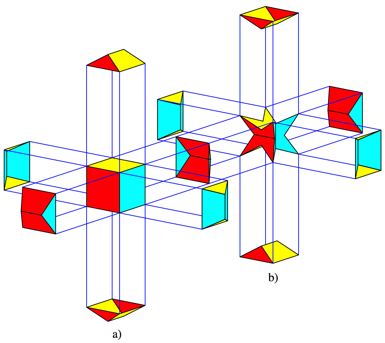Fig. 12: Hip roofs

The hip roof cut away from the cube at the right (Fig. 12b) goes to the cube at the left (Fig. 12a). We have a situation similar to the situation of anions and cations in chemistry.

A singular hip roof has the dimensions indicated in the figure 13.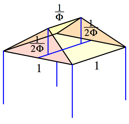Fig. 13: Dimensions of the hip roof

The notation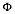means the Golden Section (Walser 2001 and 2013). The height of the roof is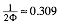.

## Eight-pointes stars

The semi-regular dodecahedron is an eight-pointed star (Fig. 14b). But it is different from the similar looking Kepler star or stella octangula (Fig. 14a).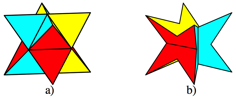Fig. 14: Kepler star and semi-regular dodecahedron

## Symmetry groups and topology

The symmetry groups of the regular and the semi-regular dodecahedra are different. The semi-regular dodecahedron has just the symmetry group S4 of the regular tetrahedron.

The regular and the semi-regular dodecahedra have the same topology (Fig. 15 and 16). Both have 20 vertices, but in the semi-regular case 12 of the vertices are hyperbolic.Fig. 15: Vertices, edges, and faces

Both have 30 edges, but in the semi-regular case six of the vertices are like the bottom of a valley. The regular dodecahedron has 12 regular pentagons as faces, in the case of the semi-regular dodecahedron the 12 faces are semi-regular pentagons.

In the diagrams of the figure 16 we see how the vertices and edges are connected. There is no difference between the two dodecahedra.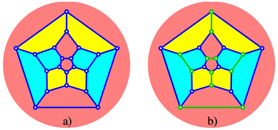Fig. 16: Diagrams for regular and semi-regular dodecahedron

## Model

We can build a paper model of the semi-regular dodecahedron (Fig. 17a).Fig. 17: Paper model

We need 6 parts according to figure 17b. We have to cut along the black lines, fold in the sense of a valley fold along the red line and in the sense of a mountain fold along the blue lines.

The red area remains visible on the outside, and the gray areas can be tucked in or glued. Hint: The model will not be very stable. Therefore I built first a model with reduced size (98%) and the visible model as second layer on it.

## Filling the space

Because of the relative situation between the two dodecahedra (Fig. 12) the regular dodecahedron can sit on the semi-regular dodecahedron like the egg on an eggcup (Fig. 18).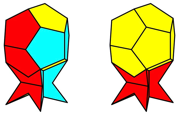Fig. 18: Eggcup

Neither the regular nor the semi-regular dodecahedra are space fillers (Coxeter 1973, p. 68f). But we can fill the space with regular dodecahedra combined with semi-regular dodecahedra (Fig. 19).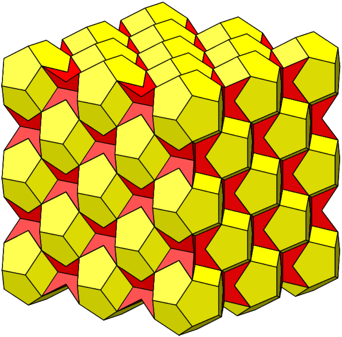Fig. 19: Filling the space

The figure 19 is somehow the spatial analogue to the figure 3.

The proof of the space-filling property is easy. First, the cube is a space filler. So we fill the space with cubes and color the cubes in black and white like a three-dimensional chessboard. Now we cut away the hip roofs from the black cubes and add them to the adjacent white cubes.

## References

Coxeter, H. S. M. (1973): Regular Polytopes. Third Edition. New York: Dover 1973. ISBN 0-486-61480-8.

Grünbaum, B. and Shephard, G. C. (1987): Tilings and Patterns. New York: Freeman. ISBN 0-7167-1193-1.

Walser, H. (2001): The Golden Section. Translated by Peter Hilton and Jean Pedersen. The Mathematical Association of America 2001. ISBN 0-88385-534-8.

Walser, H. (2013): Der Goldene Schnitt. 6., bearbeitete und erweiterte Auflage. Edition am Gutenbergplatz, Leipzig. ISBN 978-3-937219-85-1.